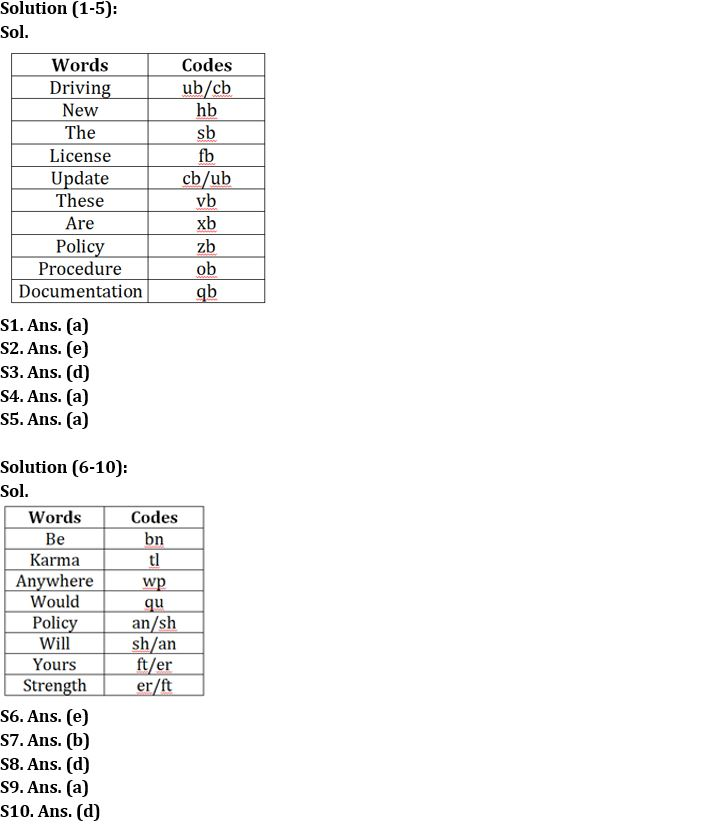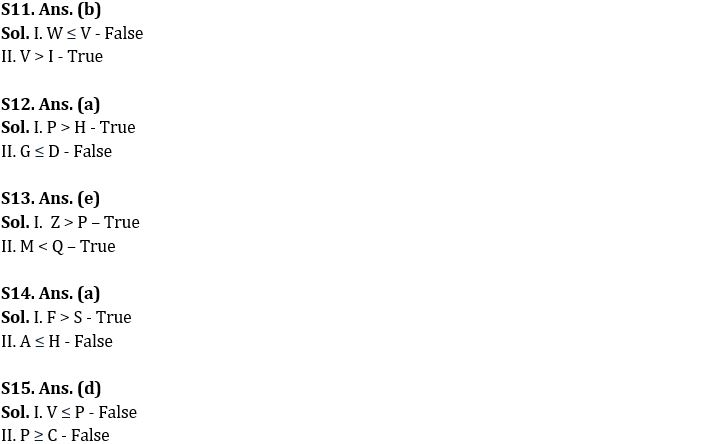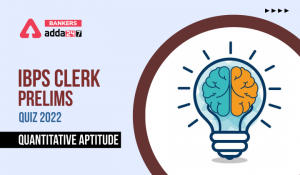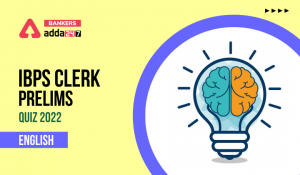Latest Banking jobs   »

# Reasoning Ability Quiz For IBPS Clerk Prelims 2022- 3rd August

Directions (1-5): Answer the following questions based on the information given below.
In a certain code of language,
“The new driving license update” is coded as “hb sb cb ub fb”
“These are policy license” is coded as “fb xb zb vb”
‘’New are procedure” is coded as “xb ob hb”
“The policy documentation” is coded as “qb sb zb”

Q1. What is the code for the word ‘these’?
(a) vb
(b) xb
(c) zb
(d) fb
(e) None of these

Q2. What is the code for the word ‘update’?
(a) ub
(b) cb
(c) hb
(d) sb
(e) Can’t be determined

Q3. The code ‘zb’ represents which of the following word?
(a) These
(b) Documentation
(d) Policy
(e) None of these

Q4. What is the code for ‘are’?
(a) xb
(b) fb
(c) ob
(d) hb
(e) None of these

Q5. What is the code for the word ‘Documentation’?
(a) qb
(b) sb
(c) zb
(d) None of these
(e) Can’t be determined

Directions (6-10): Study the following information and answer the given questions.
In coded language,
“Karma would be anywhere” is coded as “wp bn qu tl”
“Anywhere policy will be” is coded as “an wp bn sh”
“Karma be yours strength” is coded as “tl bn ft er”

Q6. What is the code for the word ‘strength’?
(a) ft
(b) er
(c) bn
(d) tl
(e) Either ft or er

Q7. If ‘will please’ is coded as ‘sh pr’ then what can be the possible code for ‘policy care’ in the coded language?
(a) an bn
(b) an ut
(c) ut wp
(d) ut tl
(e) sa pz

Q8. Which among the following word is coded as ‘bn’?
(a) Anywhere
(b) Karma
(c) Would
(d) Be
(e) None of the above

Q9. What is the code for the word ‘anywhere’?
(a) wp
(b) qu
(c) tl
(d) bn
(e) None of the above

Q10. What is the code for the word ‘Yours’?
(a) ft
(b) er
(c) sh
(d) Either ft or er
(e) None of the above

Directions (11-15): In the following questions assuming the given statement to be true, find which of the conclusion(s) among given conclusions is/are definitely true and then give your answers accordingly.

Q11. Statement: F > V ≥ S, W ≤ I < S, N < W
Conclusions: I. W ≤ V
II. V > I
(a) Only I is true
(b) Only II is true
(c) Either I or II is true
(d) Neither I nor II is true
(e) Both I and II are true

Q12. Statement: G > P ≥ B > Q, D ≥ H < Q, U > D
Conclusions: I. P > H
II. G ≤ D
(a) Only I is true
(b) Only II is true
(c) Either I or II is true
(d) Neither I nor II is true
(e) Both I and II are true

Q13. Statement: B > H > Z ≤ L < Q, Z > M > P
Conclusions: I. Z > P
II. M < Q
(a) Only I is true
(b) Only II is true
(c) Either I or II is true
(d) Neither I nor II is true
(e) Both I and II are true

Q14. Statement: F > H > V, A ≤ C ≤ V, A ≥ S
Conclusions: I. F > S
II. A ≤ H
(a) Only I is true
(b) Only II is true
(c) Either I or II is true
(d) Neither I nor II is true
(e) Both I and II are true

Q15. Statement: A > P ≥ H, C < V ≤ T < H
Conclusions: I. V ≤ P
II. P ≥ C
(a) Only I is true
(b) Only II is true
(c) Either I or II is true
(d) Neither I nor II is true
(e) Both I and II are true

Solutions#### Congratulations!Incorrect details? Fill the form again here

•Quantitative Aptitude Quiz For IBPS Cler...
•Reasoning Ability Quiz For IBPS RRB PO C...
•Quantitative Aptitude Quiz For IBPS Cler...
•Reasoning Ability Quiz For IBPS Clerk/PO...
•Quantitative Aptitude Quiz For IBPS Cler...
•English Quizzes For IBPS Clerk Prelims 2...